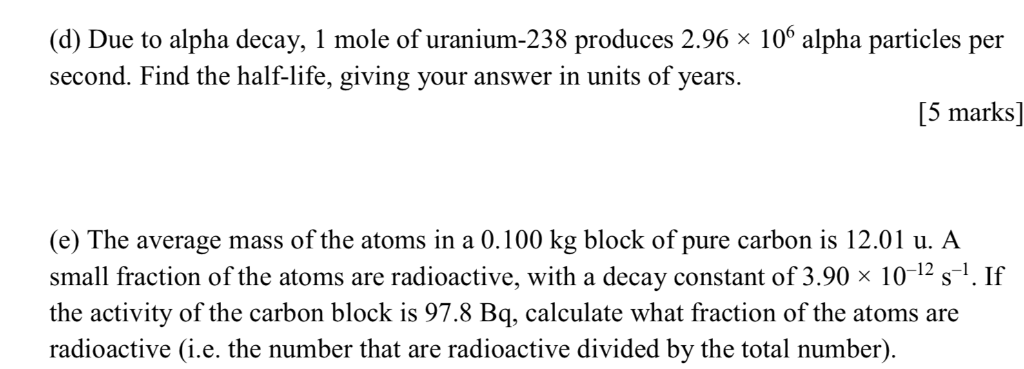# (d) Due to alpha decay, l mole of uranium-238 produces 2.96 106 alpha particles per second. Find the half-life, giving...

###### Question:(d) Due to alpha decay, l mole of uranium-238 produces 2.96 106 alpha particles per second. Find the half-life, giving your answer in units of years. [5 marks] (e) The average mass of the atoms in a 0.100 kg block of pure carbon is 12.01 u. A small fraction of the atoms are radioactive, with a decay constant of 3.90 x 10-12s. If the activity of the carbon block is 97.8 Bq, calculate what fraction of the atoms are radioactive (i.e. the number that are radioactive divided by the total number)

#### Similar Solved Questions

##### Question 16 (1 point) 1. Determine the period of the signal with units. V(t) = 80...
Question 16 (1 point) 1. Determine the period of the signal with units. V(t) = 80 cos(1000nt - 309) volts....
##### 8 § (1+-1") + 2. i=0
8 § (1+-1") + 2. i=0...
##### A system consists of the following masses loacted in the xy- plane: 5.5 kg at the...
A system consists of the following masses loacted in the xy- plane: 5.5 kg at the point (0,5), 7.0 kg at the point (3,8) and 5.0 at the point (-3, -6). Find the distance from the origin to the center of mass....
##### 14. Predict the major product of the following reaction? 15. Predict the major product of the...
14. Predict the major product of the following reaction? 15. Predict the major product of the following reaction? " " " " 16. Which of the following compounds has the longest (smallest HOMO LUMO gap)? 17. Predict the major product of the following reaction? 18. How many signals would...
##### Question 4 > You run a regression analysis on a bivariate set of data (n =...
Question 4 > You run a regression analysis on a bivariate set of data (n = 11). You obtain the regression equation y = 3.4118 + - 32.224 with a correlation coefficient of r = 0.947 (which is significant at a = 0.01). You want to predict what value (on average) for the explanatory variable will gi...
##### Question 1 of 30. How is rent received as payment for the use of real property...
Question 1 of 30. How is rent received as payment for the use of real property reported on a tax return when little or no personal services are provided? O Not-for-profit income, reported on Schedule 1 (Form 1040), line 21. O Business earned income, reported on Schedule C. O Investment income, repor...